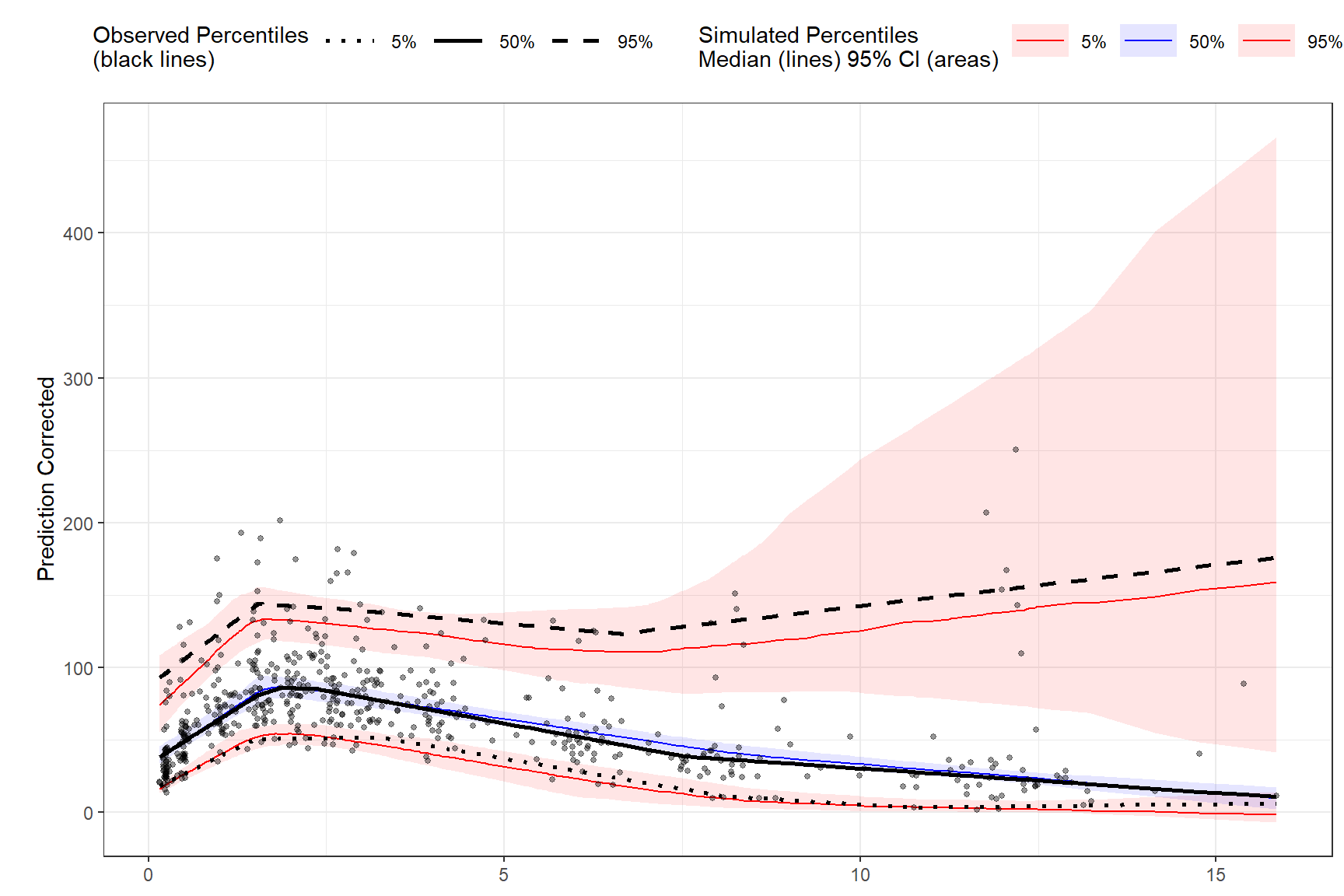# Chapter 3 Functions

## 3.1`observed()`

The `observed()` function is always the first function used in the VPC piping chain and is used to specify the observed dataset and corresponding variables. There are three arguments that are required in order to use `observed`. The first argument is either a `data.frame` or `data.table`, the second argument is the name of x-variable in the observed data, and the third argument is the name of the y-variable. Note variable names should be unquoted.

``vpc <- observed(obs_data, x = TIME, y = DV)``

## 3.2`simulated()`

The `simulated()` function is used to specify the simulated dataset and corresponding variables. There are two arguments that are required in order to use `simulated()`. Since the function is “piped” in after the `observed()` function, the first argument is the tidyvpcobj and should not be included, followed by the name of the simulated data, then the name of y-variable in the simulated data. Variable names should be unquoted and x-variable should not be included as it is recycled from the `observed()` function.

``````vpc <- observed(obs_data, x = TIME, y = DV) %>%
simulated(sim_data, y = DV)``````

## 3.3`binning()`

The `binning()` function provides the binning method to derive the vpc and should be inputted as a character string in the `bin` argument. Binning methods include: “ntile”, “pam”, “sd”, “equal”, “pretty”, “quantile”, “kmeans”, “jenks”, “centers”, “breaks”. Some methods such as “ntile” and “pam” will require you to specify the number of bins using the `nbins` argument i.e. `nbins = 9`.

If using `bin = "centers"` or `bin = "breaks` you must also provide the centers/breaks argument as numeric vector in the function i.e. `centers = c(1,3,5,7)`.

You can also bin directly on x-variable. If using this type of binning, the bin argument should be the unquoted variable name that you used in the `observed()` function i.e. `bin = NTIME` for the Nominal Time variable in the data.

Binning on x-variable, NTIME

``````vpc <- observed(obs_data, x=TIME, y=DV) %>%
simulated(sim_data, y=DV) %>%
binning(bin = NTIME)``````

Binning with “ntile”

``````vpc <- observed(obs_data, x = TIME, y = DV) %>%
simulated(sim_data, y = DV) %>%
binning(bin = "ntile", nbins = 9)``````

Binning with “breaks”

``````vpc <- observed(obs_data, x = TIME, y = DV) %>%
simulated(sim_data, y = DV) %>%
binning(bin = "breaks", breaks = c(1,5,7,9,10))``````

## 3.4`binless()`

Binless methods utilize additive quantile regression (AQR) in place of traditional binning. Use the `binless()` function instead of `binning()` to derive a binless VPC. By default, `binless()` performs AQR at the 5%, 50%, and 95% quantiles but you can change this using the `qpred` argument which takes a numeric vector of length 3 i.e. `qpred = c(.1, .5, .9)` for the 10%, 50%, 90% quantiles.

The lambda smoothing parameters for each quantile are optimized by default with AIC as indicated by the `optimize = TRUE` argument, however, if you would like to use different lambda values for each quantile set `optimize = FALSE` and specify lambda values for each quantile as a numeric vector of length 3 with the `lambda` argument i.e. `lambda = c(1,3,2)` corresponds to the lambda values for the quantiles in the `qpred` argument (lower, median, upper). Note: the higher the lambda value the smoother fit to the data.

Binless optimized

``````vpc <- observed(obs_data, x=TIME, y=DV) %>%
simulated(sim_data, y=DV) %>%
binless()``````

Binless with user specified lambda values at 10%, 50%, 90% quantiles. Set `optimize = FALSE` and provide lambda smoothing parameters as a vector of length 3 for lower, median, upper quantiles.

``````vpc <- observed(obs_data, x=TIME, y=DV) %>%
simulated(sim_data, y=DV) %>%
binless(qpred = c(0.1, 0.5, 0.9), optimize = FALSE, lambda = c(1,3,2))``````

## 3.5`vpcstats()`

The `vpcstats()` function is always the final function used in the piping chain and calculates the statistics needed to plot a VPC. If using `binning()`methods, you may specify alternative quantiles using the `qpred` argument. The default quantiles used are 5%, 50%, 95% i.e. `qpred = c(0.05, 0.5, 0.95)`. If using `binless()` method, there is no need to include the `qpred()` argument here as the quantile values supplied in the `binless()` function will be used to calculate the VPC statistics for plotting.

``````vpc <- observed(obs_data, x=TIME, y=DV) %>%
simulated(sim_data, y=DV) %>%
binning(bin = NTIME) %>%
vpcstats()

plot(vpc)``````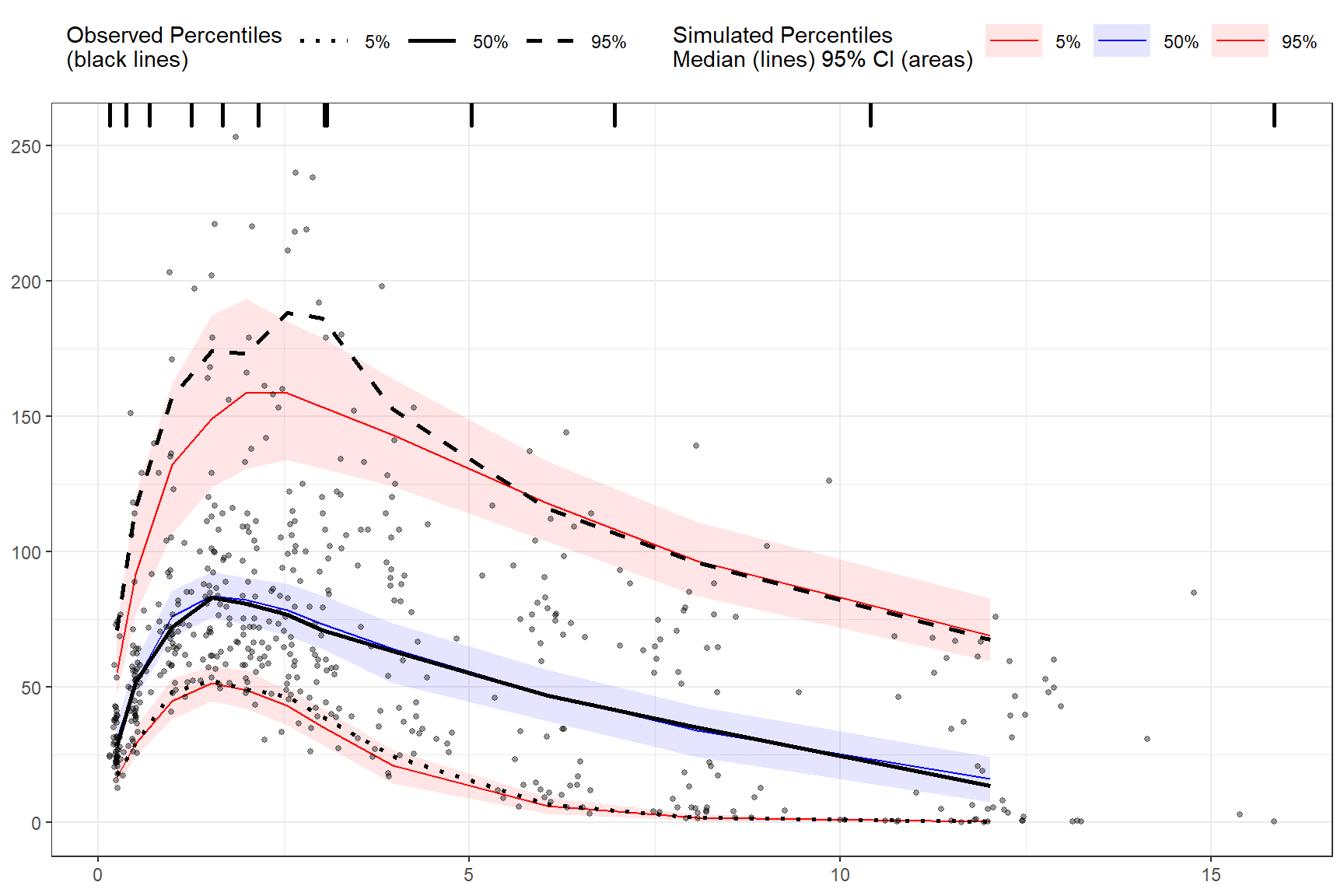## 3.6`stratify()`

To stratify VPC include the `stratify()` function before using the `binning()` or `binless()` function and use the unquoted stratification variable(s) name as a formula. Let’s stratify on `GENDER` in the data, which contains 2 levels (GENDER = “M”, GENDER = “F”). Include as many stratification variables as your model calls for.

``````vpc <- observed(obs_data, x=TIME, y=DV) %>%
simulated(sim_data, y=DV) %>%
stratify(~ GENDER) %>%
binning(bin = "pam", nbins = 7) %>%
vpcstats()

plot(vpc)``````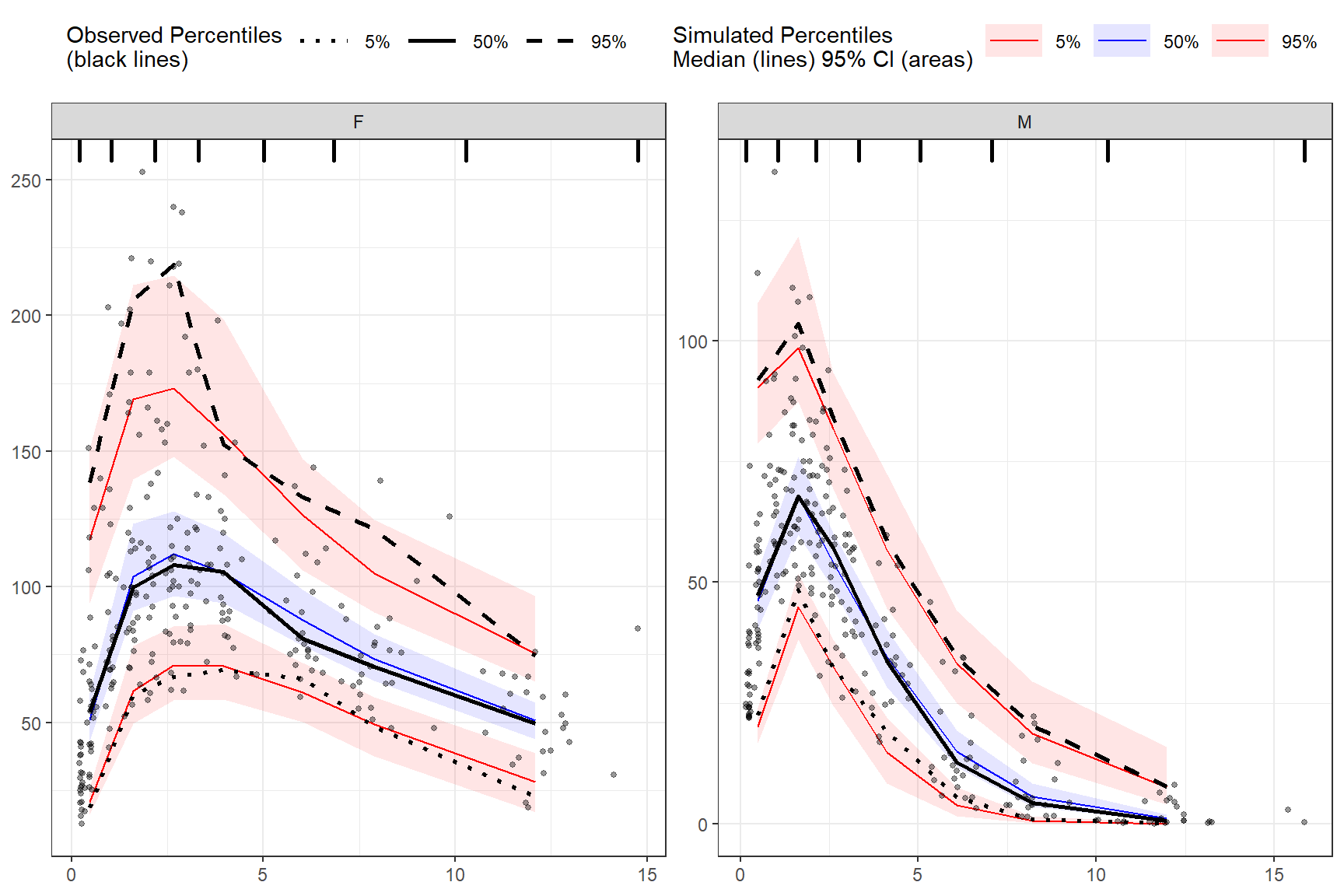Using multiple stratification variables `GENDER` and `STUDY`.

``````vpc <- observed(obs_data, x=TIME, y=DV) %>%
simulated(sim_data, y=DV) %>%
stratify(~ GENDER + STUDY) %>%
binless() %>%
vpcstats()

plot(vpc)``````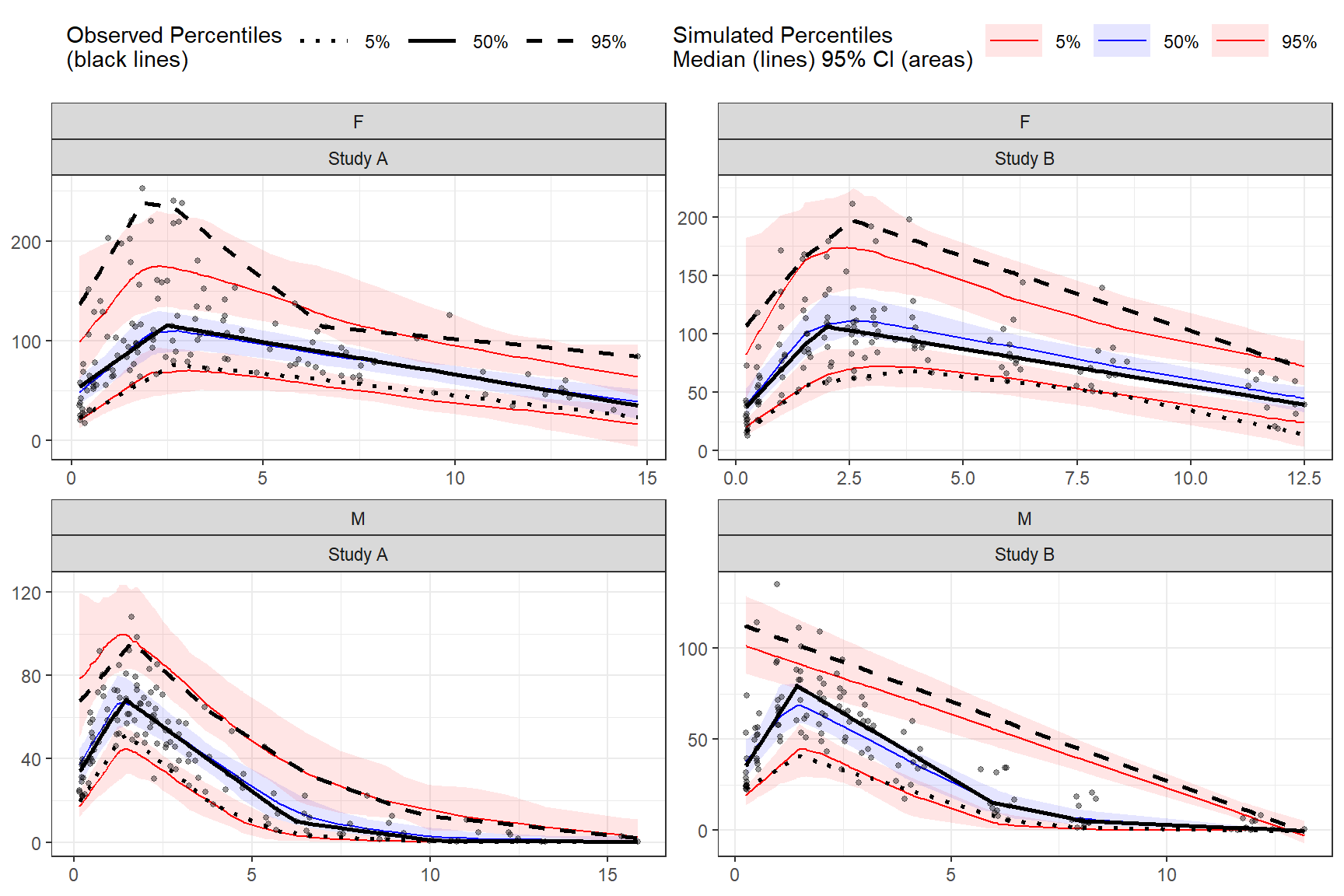## 3.7`censoring()`

To censor observed data below lower limit of quantification (LLOQ), include the `censoring()` function after `simulated()` and use the `lloq` argument to specify either a variable in the data or specific value for censoring. The `blq` argument creates a logical TRUE/FALSE in the data that indicates whether the value is below the limit of quantification and is typically defined as rows with DV < LLOQ in the data. Using the `censoring()` function will censor only observed data below lower limit of quantification when plotting, simulated data will still be plotted.

Censoring using numeric value.

``````vpc <- observed(obs_data, x=TIME, y=DV) %>%
simulated(sim_data, y=DV) %>%
censoring(blq=(DV < 25), lloq=25) %>%
binning(bin = "jenks", nbins = 5) %>%
vpcstats()

plot(vpc)``````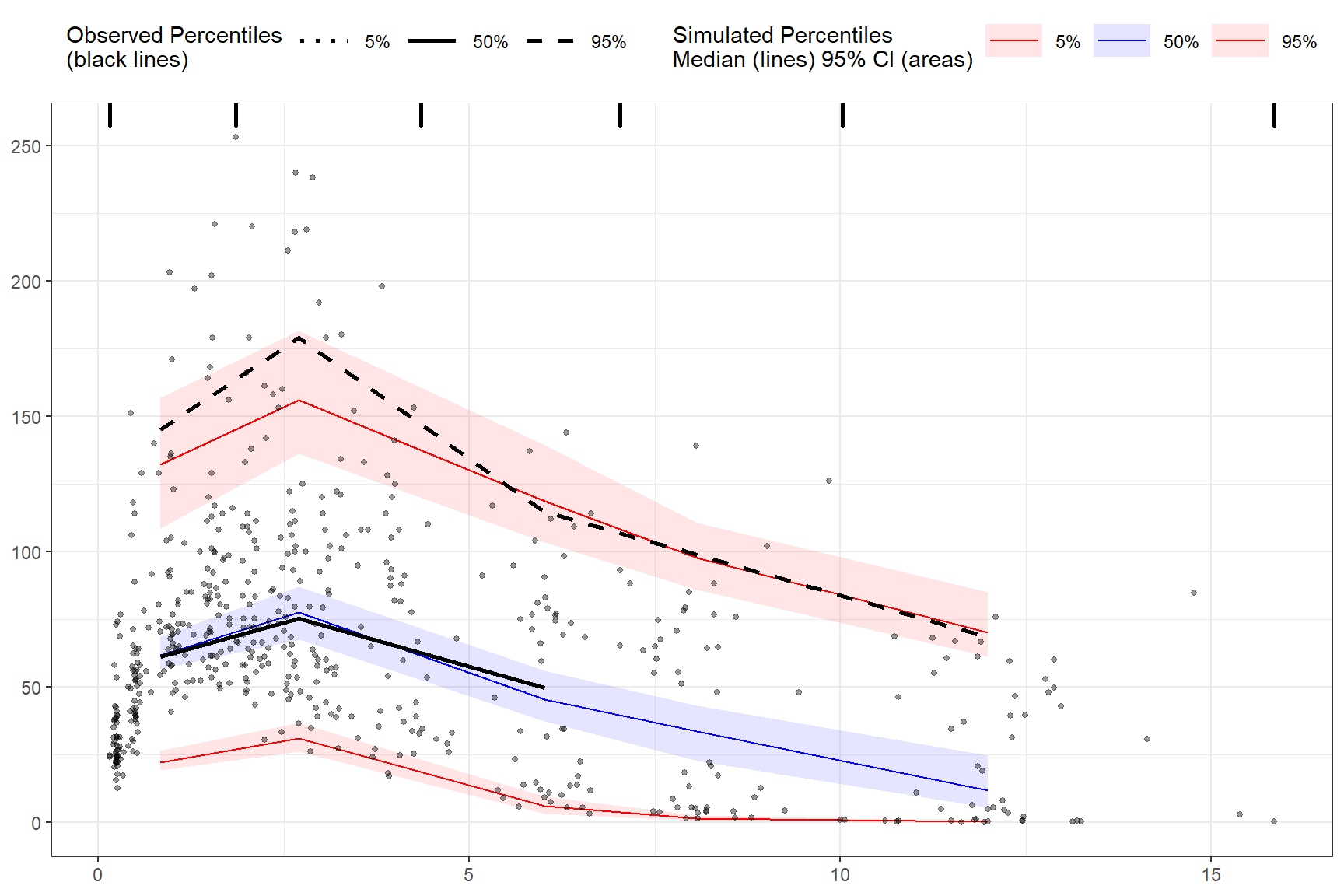Censoring using `LLOQ` variable in the data.

First, let’s add an LLOQ variable to the observed data.

``obs_data\$LLOQ <- 50``

Then we’ll specify lower limit of quantification as the unquoted variable name in our data `LLOQ`. Let’s also provide our own lambda values.

``````vpc <- observed(obs_data, x=TIME, y=DV) %>%
simulated(sim_data, y=DV) %>%
censoring(blq=(DV < LLOQ), lloq=LLOQ) %>%
binless(optimize = FALSE, lambda = c(1.5, 2.5, 1.7)) %>%
vpcstats()

plot(vpc)``````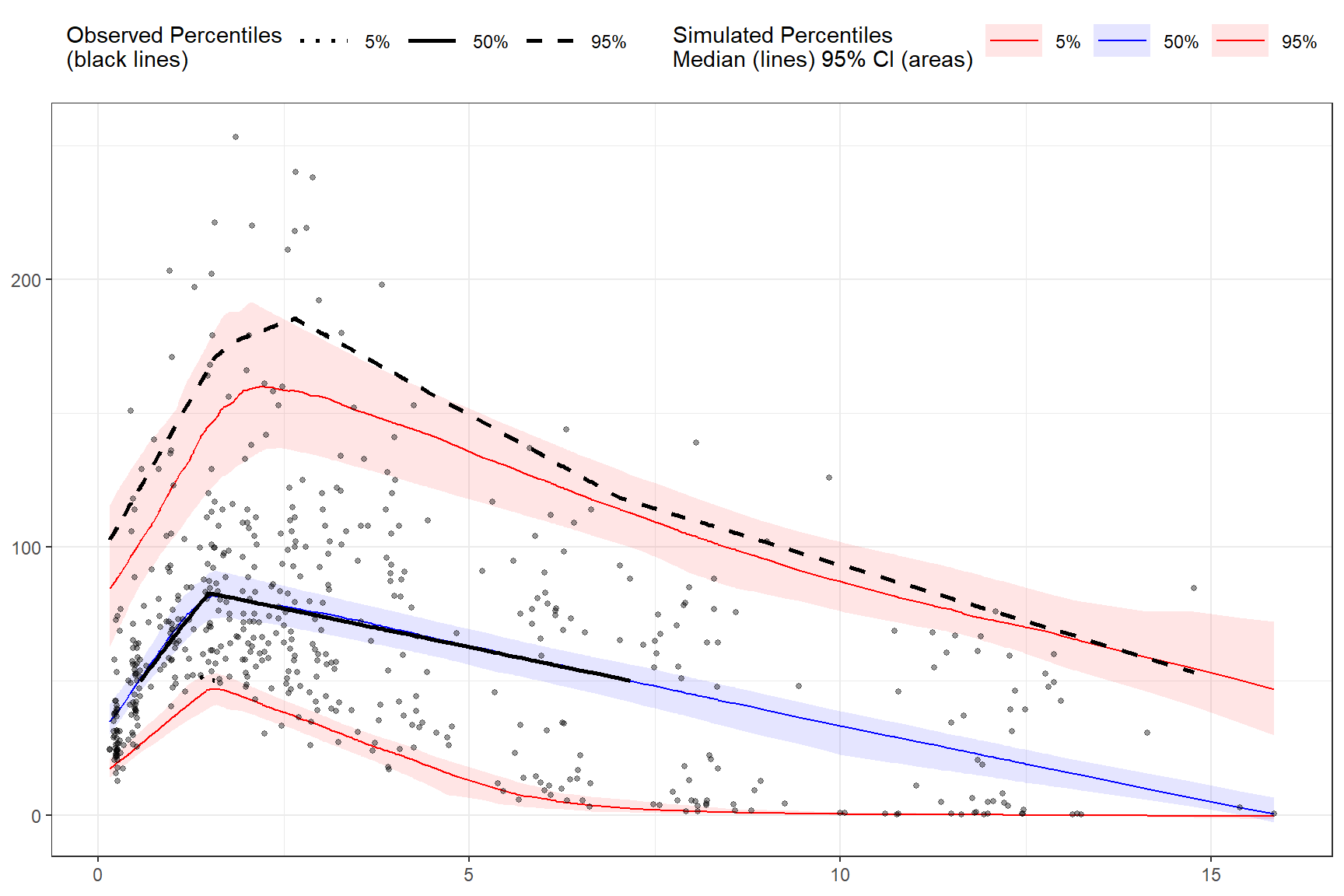The `tidyvpc` package also allows you to use different LLOQ for each level of stratification variable. We’ll set an `LLOQ` value of `50` for `Study A` and `25` for `Study B` and calculate statistics at 5%, 50%, 90% quantiles.

``````obs_data\$LLOQ <- ifelse(obs_data\$STUDY == "Study A", 50, 25)

vpc <- observed(obs_data, x=TIME, y=DV) %>%
simulated(sim_data, y=DV) %>%
censoring(blq=(DV < LLOQ), lloq=LLOQ) %>%
stratify(~ STUDY) %>%
binning(bin = "pam", nbins = 4) %>%
vpcstats(qpred = c(0.1, 0.5, 0.9))

plot(vpc)``````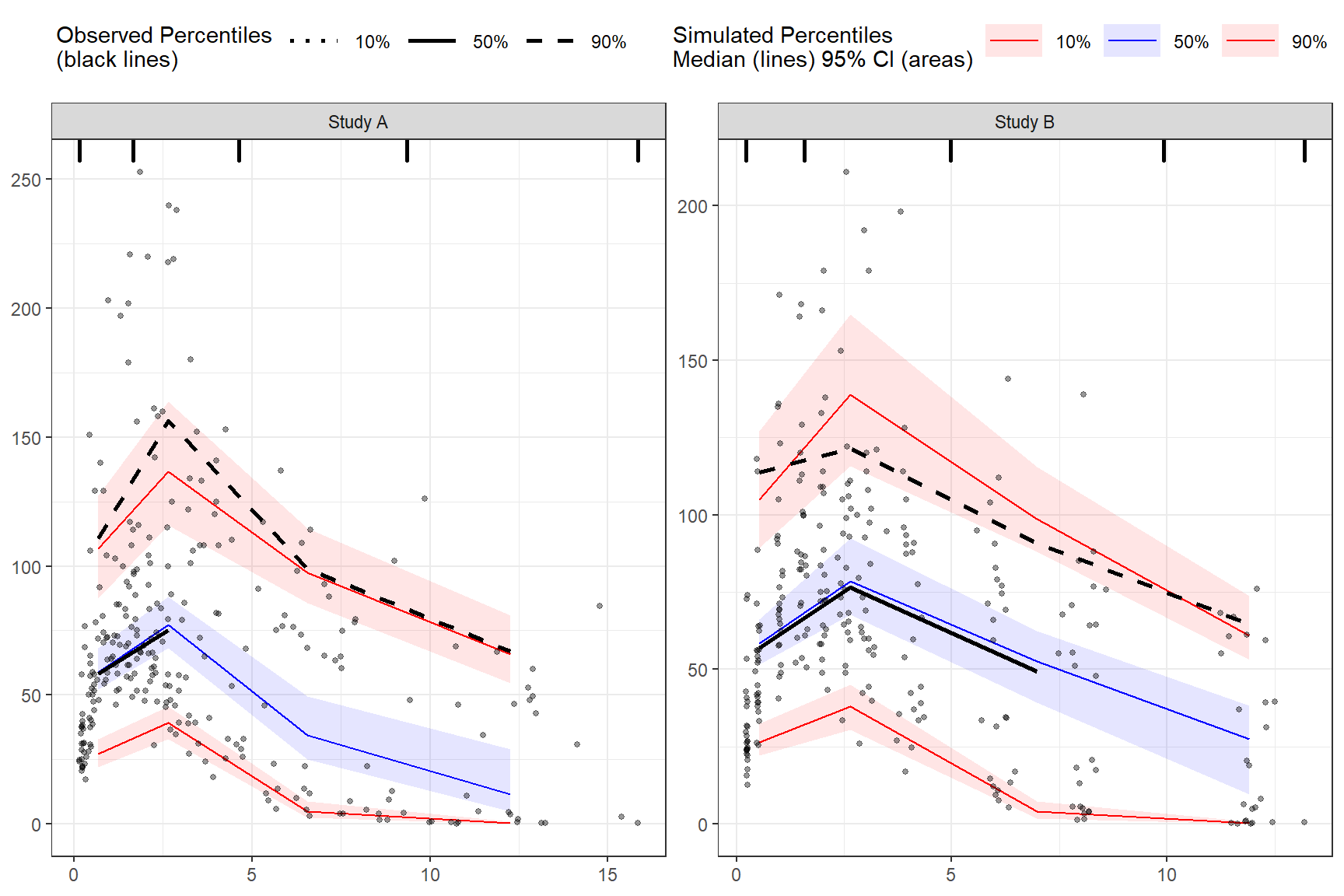## 3.8`predcorrect()`

To derive a prediction corrected VPC (pcVPC) use the `predcorrect()` function. The `predcorrect()` function takes one required argument `pred` which should be the unquoted variable name of the population prediction variable in the data. If using binning methods, the `predcorrect()` function should be called after `binning()`, however, if performing LOESS pcVPC for `binless()` methods, use `predcorrect()` before calling `binless()` and set `binless(loess.ypc = TRUE)`. Note: if model was fit using log scale of DV make sure to include the argument `log = TRUE` in `predcorrect()` to perform the appropriate prediction correction calculation.

Prediction corrected using binning methods.

``````vpc <- observed(obs_data, x=TIME, y=DV) %>%
simulated(sim_data, y=DV) %>%
stratify(~GENDER) %>%
binning(bin = NTIME) %>%
predcorrect(pred=PRED) %>%
vpcstats()

plot(vpc)``````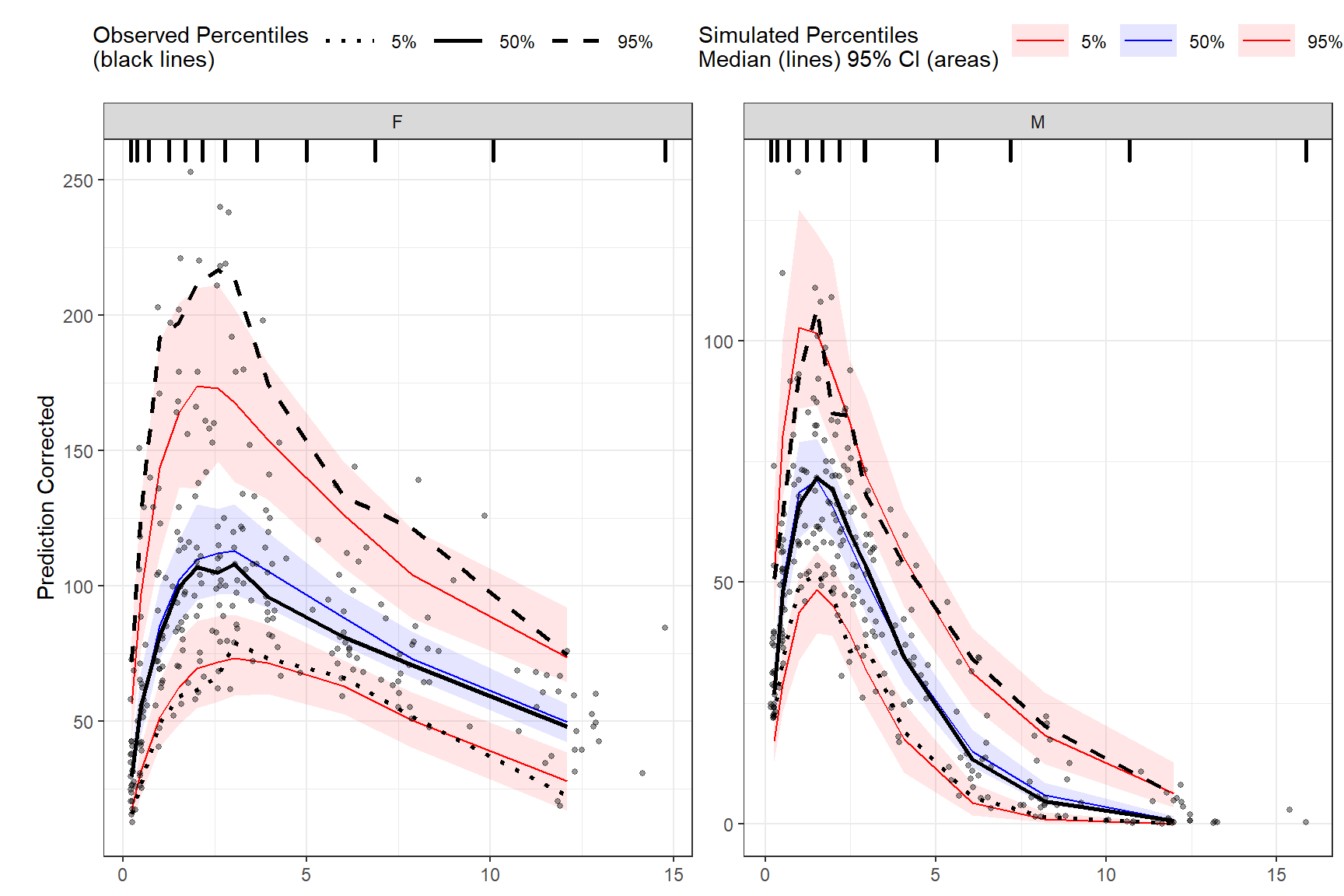LOESS prediction corrected using binless method for 10%, 50%, 90% quantiles. If `optimize = TRUE`, the LOESS smoothing parameter, span, will be automatically optimize using AIC. Note: `predcorrect()` must be called before `binless()` if setting `loess.ypc = TRUE`.

``````vpc <- observed(obs_data, x=TIME, y=DV) %>%
simulated(sim_data, y=DV) %>%
stratify(~GENDER) %>%
predcorrect(pred=PRED) %>%
binless(qpred = c(0.1, 0.5, 0.9), optimize = TRUE, loess.ypc = TRUE) %>%
vpcstats()

plot(vpc)``````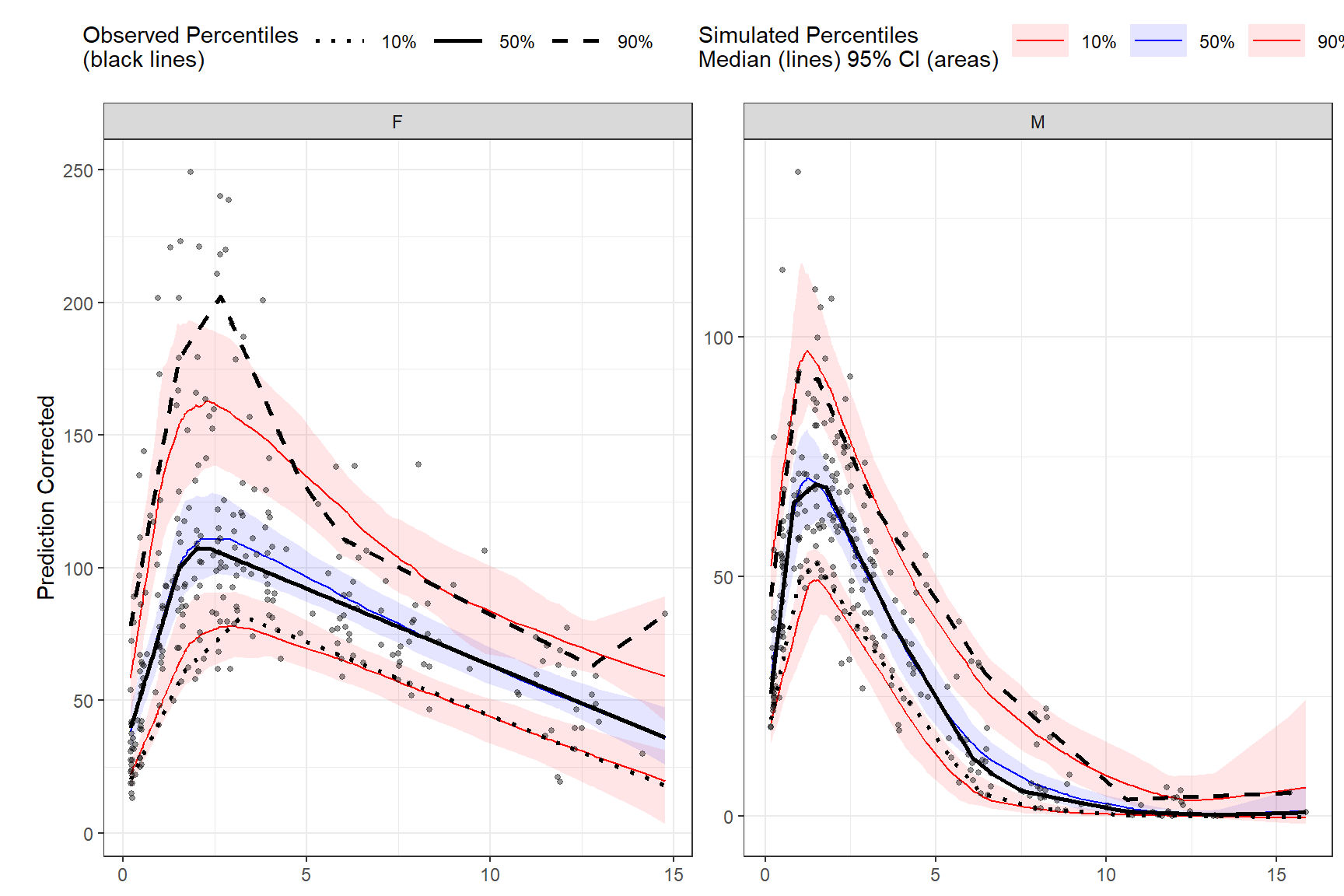To specify your own span value LOESS pcVPC instead of optimizing with AIC, use the `span` argument in the `binless` function. Span should be a numeric between [0,1], with higher values providing a smoother fit. Remember, to also include the smoothing parameters for AQR by using the `lambda` argument and set `optimize = FALSE`.

``````vpc <- observed(obs_data, x=TIME, y=DV) %>%
simulated(sim_data, y=DV) %>%
predcorrect(pred=PRED) %>%
binless(optimize = FALSE, lambda = c(.95,3,1.2), loess.ypc = TRUE, span = .6) %>%
vpcstats()

plot(vpc)``````